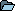ContinuousWave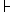WhalerModerated Discussion AreasContinuousWave: Small Boat ElectricalRadio Propagation Over Water
 Author Topic:   Radio Propagation Over Water jimh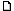posted 05-02-2013 10:11 AM ET (US)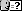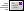For some time I have been looking for a good model or basis for estimating path loss of radio signals over water. I found some data about this in the Federal Communication Commission (FCC) regulations for PART 80--STATIONS IN THE MARITIME SERVICE. The commission has provided some charts for the purpose of calculating the coverage of a shore station. A chart showing path loss appears in 80.767 Propagation Curve. For convenience, just the chart is available fromThe chart is very interesting because it shows signal loss with distance with several curves. There is a curve labeled "Free Space", which represents the theoretical best-case loss curve for a radio wave traveling a certain distance. There are also curves for real-world situations, with various antenna heights.The curves show that for very short distance paths and very tall antennas, the path loss in the real world is the same as in free space, but once the path distance becomes more than a few miles, the characteristics of the path loss change remarkably.I investigated this chart to see if I could deduce the path loss factor for the distance that was being used. I will describe my method.First, I looked closely at the free-space curve. I found the signal levels (dBu) for the distances (d) of 10 and 100 miles. ` d dBu 10 83 100 63`When the distance changed by a factor of ten, the signal decreased by -20dB. This suggests the relationship isdB = x log(d)-20 = x log(10)x = -20 / log(10) = -20The curve on the chart seems to show that path loss as a function of distance behaves according to the relationshipdB = -20 log(d)This is exactly what would be expected. The factor -20 log(d) is used in the free-space model of path loss. Next, I investigated the other curves to see what sort of relationship between signal level and distance they represented. Using the curve for an antenna of 100-feet, I found the following values of signal and distance:` d dBu 5 75 50 19`When the distance changed by a factor of ten, the signal decreased by -56dB. This suggests the relationship isdB = x log(d)-56 = x log(10)x = -56 / log(10) = -56The curve on the chart seems to show that path loss as a function of distance behaves according to the relationshipdB = -56 log(d)A relationship between signal and distance where the path loss increases at that rate is significantly more lossy than some of the other estimates I have seen. In another article,Estimating Path Loss on Marine Non-Line-of-Sight Pathsthe path loss factor was found to be often cited in the range of -40 log(d) to perhaps -46 log(d), but not nearly as steep as the -56 log(d) suggested by the FCC propagation chart.One possible explanation for the very high estimate of path loss in the FCC chart is to create a very conservative estimate of coverage area. The chart is part of a procedure to establish the coverage area of shore stations, so the intent may be to use a method that will result in a coverage area that is very reliable. This may explain why the propagation loss for distance is predicted to be so rapid compared to other models. jimhposted 05-02-2013 11:55 AM ET (US)In my earlier article (hyperlinked above) I estimated the path loss based on my estimate of the signal level received for a particular station, WXN69. I estimated the signal level received was -97dBm. This was just a rough estimate, based on the receiver having a rated sensitivity for a 10dB SINAD of -107 dBm, and judging (by ear and experience) the received signal to be perhaps 10dB stronger than that minimum signal level. If the signal level I observed were actually at the -107dBm level, then the path loss equation derived from that estimate would have needed a distance factor of -51.6 log(d) for (d) in miles. That value is not too far off from the FCC chart curve's behavior, which seems to suggest -56 log(d) is the appropriate model.It is clear that the free-space model estimate is not appropriate unless the path is very short and there is a lot of ground clearance (to eliminate the influence of the ground and any reflections). It is also clear that any estimate of the effect of distance on path loss that suggests it will less the -40 log(d) is much too optimistic and probably completely unrealistic. jimhposted 05-03-2013 11:44 AM ET (US)I am sure that at this point some readers--if there are any readers at all--are probably wondering what I am talking about. Let me explain a bit more in detail.When the signal loss or path loss for a certain distance is described as being 20 log(d)we are saying that the path loss increases with distance accord to that rate. Let us explore the increase in path loss when the path length doubles, as in a path of 10-miles increasing to a path of 20-miles. The path loss due to distance will be:For 10-milesloss = 20 log(10)loss = 20dBFor 20-milesloss = 20 log(20)loss = 26dBWhen the path distance doubled, the path loss changed 6dB. Because there was decrease in signal, we say the path loss changed -6dB The notation -6dB represent a ratio. In terms of a ratio of power, we can find the power ratio from the definition of dB for power, which isdB = 10 log (P1/P2) where P1, P2 are two power levelsWe can also evaluate that relationship to find the power ratio represented by the dB expression. That becomes(P1/P2) = 10^(dB/10) Now we evaluate for -6dB to find the ratio of powers:(P1/P2) = 10^(-6/10)(P1/P2) = 10^-0.6(P1/P2) = 0.25In other words, when the path distance doubled, the signal power decreased to 0.25 of the original power, or one-fourth the original power. This is another way of describing the inverse square law relationship. I think many readers will be familiar with that behavior, but may have not realized that it was represented in the notation 20 log(d).When models for propagation use a factor for distance of 20 log(d), they are assuming that the decrease in signals will just obey a simple inverse square law relationship. This is the behavior that would occur in free space, an ideal environment in which nothing else affects the radio wave as it propagates. In the real world there are other factors in the environment that affect the signal loss in propagation, causing the real-world signal decrease to be greater. This behavior is reflected in having a path loss model in which the distance factor is greater than 20 log(d). jimhposted 05-08-2013 07:55 AM ET (US)Investigating more into path loss models, I came across a reference to the c.1957 work of Egli, who proposed that path loss could be calculated by the relationship ofPr = 0.66*Gb*Gm * [(Hb*Hm)^2/d^4] *[40/f]^2 *PtwherePr = received powerPt = transmit powerGm= gain of mobile antenna (not in dB)Gb = gain of base antenna (not in dB)Hm =height of mobile antenna (meters)Hb = height of base antenna (meters)d = path distance (meters)f = MhzThis model contains several significant observations--the path loss increases at a rate of 40 log(d) (from the 1/d^4 factor)--there is decrease in path loss with antenna height at a rate of 6dB per octave, that is, doubling the height improves the received signal by a factor of four;--and frequency influences these effects. There is an empirical factor for frequency which is applicable for frequencies in the range of 30-MHz to 1,000-Mhz, and for distances in the range of 1 to 50-km. For more on Egli's model, and propagation path loss models in general, see jimhposted 05-08-2013 09:18 AM ET (US)Egli's model is not very optimistic about a typical boat-to-boat path. Using the following inputAntenna gain = 2 for both antennas, i.e. 3-dBAntenna height = 3-meters, i.e. about 10-feet, for both antennasTransmitter power = 25-wattsFrequency = 156-MHzthe model calculates the received signal power as followsMiles__dBm0.6 = -94.51.2 = -106.52.4 = -119.65.0 = -130.6As you can see, when the distance doubles the path loss increases by 12dB, which is what is suggested by the 40 log(d) factor.A really good receiver sensitivity is 0.25-microVolt, which is a signal level of -119dBm. Egli's model suggests that we will reach that signal level at a range of only 2.5-miles. The model does much better when antenna height is increased. For example, if one antenna is raised to 10-meters (33-feet), then the path distance for a -119dBm received signal doubles to 5-miles.The model is for propagation over smoothly rolling terrain with about 50-foot variation, so calm seawater will probably be better, that is, lower path loss. The model is also statistical, that is, it predicts a path loss that occurs about half the time. jimhposted 05-08-2013 09:45 AM ET (US)I also found this on-line calculator that is said to use the Egli model:I built my own spreadsheet version of an Egli's calculator, and it shows very good agreement with the one above if the 0.66 factor is removed. That factor was probably to account for statistical probabilities. jimhposted 05-12-2013 10:34 AM ET (US)Using the on-line calculator based on Egli's model (linked above), the path loss for the following situation Antenna Height-1 = 100-feet (e.g., a shore station)Antenna Height-2 = 10-feet (e.g., a small boat)Frequency = 157-MHzTransmission distance = 30-milesis calculated to be -160dB. We can now predict the signal level we might have for this path if we have the following characteristics in each station:Transmitter power = 25-WattsAntenna gain = 3dBA transmitter power of 25-Watts is a signal of +44dBm. Both the transmit antenna and the receive antenna have 3-dB gain. We can now predict the received signal level as follows:TxPower + TxAntGain + RxAntGain +PathLoss = RxPower+44dBm + 3dB + 3dB + -160dB = -110dBmA received signal level of -110dBm in a 50-Ohm system corresponds to a 0.7-microVolt of signal. Most modern VHF Marine Band radios have receivers with sensitivity of 0.5-microVolt for 10-dB SINAD, so the received signal on this path should be above the receiver's rated sensitivity.For the path loss, transmitter power, antenna gains, and receiver sensitivity described above, the Egli's model predicts a useable signal at a range of 30-miles. Egli's model is based on gently rolling terrain over land with hills not more than 50-feet high. On an all-water path with no intervening terrain, the Egli's model should be a reasonably accurate predictor of radio coverage, especially over saltwater and calm seas. contenderposted 05-12-2013 01:23 PM ET (US)So, basically, if you venture further out than 30 miles, you should carry a satellite phone if you want to stay in contact with land. jimhposted 05-12-2013 06:47 PM ET (US)I suppose you could make an inference like that. The reliable range depends on the particular path and the particular stations. jimhposted 05-14-2013 01:46 PM ET (US)As I noted above, the Egli's model includes an allowance for antenna height that is rather generous because every time the height is doubled the path loss decreases by 6-dB. Another way to say this is that doubling the height causes the signal to increase by a factor of four.I inspected the FCC chart of path predicted coverage (linked above) and found that the same behavior was used in their predictive model: when the antenna height is doubled the predicted signal increases by 6-dB.There seems to be some agreement in the two models on the influence of antenna height, and that influence seems really very generous. It is my understanding that both of these models are adaptations of real measurements of signal transmission over real terrain in the VHF and UHF spectrum, that is, they are not theoretical approaches but rather embodiments of actual observed phenomenon which have been then reduced to an empirical formula. The influence of antenna height seems very significant in these two methods of predicting radio propagation, and this seems to suggest that antenna height really is very important, particularly when it is possible without too much mechanical difficulty to create a doubling of the antenna height, as would occur, say, if an antenna were raised to 10-feet from 5-feet in height. There is evidence in both the FCC data and Egli's data that such an antenna height increase will pay back a substantial dividend in increased signal (and thus increased range).On this basis, it seems that one ought to prefer height to antenna gain in most instances. For example, it would be better to raise an antenna of 3-dB gain to double the height of an antenna of 6-dB gain. The increase in height is predicted to return a 6-dB increase in gain over most signal paths, while the increase in antenna gain would only return 3-dB (and then only in the main lobe which must be pointed at the distance station to achieve the gain).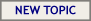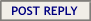Hop to: ContinuousWave: The Whaler GAM or General AreaContinuousWave: Whaler MarketplaceContinuousWave: Whaler Repairs/ModsContinuousWave: Whaler PerformanceContinuousWave: Cetacea CommentsContinuousWave: Small Boat ElectricalContinuousWave: Trips and RendezvousContinuousWave: Post-Classic Whalers